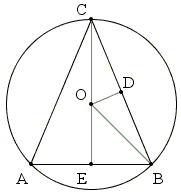Maximum Area Property of Equilateral Triangles

A particular case of the Isoperimetric Theorem tells us that among all triangles with the same perimeter, the equilateral one has the largest area. A related theorem concerning the triangles inscribed into a given circle is also true:

Among all triangles inscribed in a given circle, the equilateral one has the largest area.

Proof

Among all triangles inscribed in a given circle, the equilateral one has the largest area.

Proof

The proof depends on the following

Lemma

Among all triangles inscribed in a given circle, with a given base, the isosceles one has the largest area.

The assertion of the lemma is quite obvious:Among all inscribed triangles with a given base, the tallest one is isosceles and, therefore, it has the largest area, due to the standard formula A = b×h/2, where A, b, and h are the area, the base and the altitude of a triangle.

The lemma shows that for a triangle that has two unequal sides, there is another triangle (an isosceles one at that) with the same circumcircle but larger area. The only triangle for which no improvement is possible is equilateral.

The lemma also shows that in order to prove the statement we only need to look among isosceles triangles. Consider the diagram below:Let α be the base angle of an isosceles triangle ABC. Then half the apex angle at C equals 90° - α. Let O be the circumcenter of the triangle and D and E the midpoints of sides BC and AB, respectively. Thus, in the diagram, we successively obtain

∠ACB = 180° - 2α,
∠BCE = ∠OCD = 90° - α,
OB = OC, BD = CD,
∠OBD = ∠OCD = 90° - α,
∠OBE = α - (90° - α) = 2α - 90°,90° - 2α,2α - 90°,2α
OE = R sin(2α - 90°) = R -cos,sin,-cos,cos,tan(2α),
CE = R(1 - cos(2α)),
AB = 2 EB = 2R cos(2α - 90°) = 2R sin,sin,cos,tan(2α),
Area( ΔABC) = AB×CE / 2 = R² (1 - cos(2α)) sin(2α).

So to maximize the area of triangle ABC we need to find the maximum of function f(β) = sin(β)(1 - cos(β)), where β = 2α. The simplest way to do that is to compute and equate to 0 the derivative:

f'(β) = cos(β)(1 - cos(β)) + sin(β) sin(β) = -cos²(β) + cos(β) + (1 - cos²(β)).

Letting x = cos(β) we are left with solving the quadratic equation f(x) = 2x² - x - 1 = 0. Using the quadratic formula, the equation has two roots: x = -1/2 and x = 1, the latter being a spurious byproduct of the applied technique, i.e., looking for the roots of a derivative. Derivatives vanish at internal extrema and saddle points, whereas, for this problem we are only interested in a maximum. In the range of interest (0 < β < 180°), the equation cos(β) = -1/2 admits only one solution, viz., β = 120°, hence 2α = 120°, and α = 60°, making ΔABC indeed equilateral,scalene,equilateral,right,obtuse.

(The second root x = 1, leads to cos(2α) = 1. Allowing for an expanded angle range that includes 0, α = 0. A triangle with a 0° angle is called degenerate. Its area is 0 and, therefore, it serves an example of an inscribed triangle with the least area.)

It goes without saying (see the discussion of the general Isoperimetric Theorem) that our statement admits an equivalent formulation:

Among all triangles with the given area, the equilateral one has the smallest circumscribed circle.• Equilateral Triangles on Sides of a Quadrilateral
• Euler Line Cuts Off Equilateral Triangle
• Four Incircles in Equilateral Triangle
• Problem in Equilateral Triangle
• Problem in Equilateral Triangle II
• Sum of Squares in Equilateral Triangle
• Triangle Classification
• Isoperimetric Property of Equilateral Triangles
• Angle Trisectors on Circumcircle
• Equilateral Triangles On Sides of a Parallelogram
• Pompeiu's Theorem
• Circle of Apollonius in Equilateral Triangle
•